Published: 30 June 2017

# Experimental and numerical simulation studies of the squeezing dynamics of the UBVT system with a hole-plug device

Wen-bin Gu1
Yun-hao Hu2
Zhen-xiong Wang3
Jian-qing Liu4
Xiao-hua Yu5
Jiang-hai Chen6
Jing-lin Xu7
1, 2, 3, 4, 5, 7PLA University of Science and Technology, Nanjing, China
5Unit No. 73198 of PLA, Nanjing, China
6Unit No. 65307 of PLA, Jilin, China
Corresponding Author:
Wen-bin Gu
Views 28

#### Abstract

In this paper, a method is proposed to secure an autonomous underwater blasting vibration test (UBVT) system with plugs to deep-water rock, and its specific configuration concept and plugging principle are illustrated. Using the principle of statics, a mathematical model is established for the squeezing force in the process of pressing the hole-plug device (HPD) into holes in rocks. The tension-compression test is conducted on the plugs in round granite holes to obtain the axial pressure-displacement curves of the pressing process with the HPD spring parameter $K$, friction coefficient $\mu$ between the HPD and the rock-wall, and the dynamic contact friction attributes between the metallic HPD and the rock-wall of hole in granite. The axial pressure with such parameters as $K$, $\mu$, and the squeezing velocity $v$, among others, and the four steps of the pressing process are numerically simulated. The relations of the characteristic squeezing force with $K$, $\mu$, and $v$, as well as the mechanisms of these parameters that influence HPD usage and the sensitivity coefficient, are revealed. The findings of the present study provide references for setting the HPD configuration parameters and for formulating plug-specific construction methods.

## 1. Introduction

Seismic waves generated by deep-water rock blasting may damage the safety of underwater structures and facilities, and their deep-water propagation and hazardous effects have become problems that remain to be solved in this field [1-6]. The characteristics of underwater blasting and the propagation laws of induced vibration are both different from those of land blasting due to the inﬂuence of underwater environments such as water mass, water-saturated sediments, and rock mass [7, 8]. Limited by underwater blasting vibration test (UBVT) techniques and means, current study of underwater blasting vibration mainly concentrates on the response of land ground and structures, deep-water rock blasting vibration effects have been rarely reported at home and abroad [9-11]. We invented an UBVT system that integrates vibration signal acquisition and storage, and multiple autonomous triggering, among others . By comparing the calibration, metering, and land blasting vibration test results, the precision and accuracy of the UBVT system is validated [13, 14]. The UBVT system provides technical support for testing the underwater blasting vibration effects, and the method of securing the test system to seabed rock offers engineering support. To solve problems of securing the test system to seabed rock and to ensure no relative displacement occurs between the system and the underwater measuring point under the blasting vibration effects, we presented methods of plugging the test system into underwater rock with holes .

## 2. Mathematic model of axial force analysis for pressing HPD into holes

Force analysis of pressing into the round rock hole is shown in Fig. 1, and the relative axial displacement between the plugs of different layers is presented in Fig. 2.

Force analysis is conducted at different steps of the pressing process, and the corresponding statics equations are established at different steps of the pressing process. The following expressions of axial pressure using the four steps are obtained:

1
${F}_{c}\left(z\right)=\left\{\begin{array}{lll}\frac{{n}_{1}K\left[\mathrm{\Delta }{x}_{0}+g\left(z\right)-{l}_{0}\right]\left[\mu g\left(z\right)+\left({d}_{0}-z\right)\right]}{g\left(z\right)-\mu \left({d}_{0}-z\right)},& & \\ {n}_{1}\mu K\mathrm{\Delta }{x}_{1},& & \\ {n}_{1}\mu K\mathrm{\Delta }{x}_{1}+\frac{{n}_{2}K\left[\Delta {x}_{0}+g\left(z-{z}_{1}\right)-{l}_{0}\right]\left[\mu g\left(z-{z}_{1}\right)+\left({d}_{0}-z+{z}_{1}\right)\right]}{g\left(z-{z}_{1}\right)-\mu \left({d}_{0}-z+{z}_{1}\right)},& & \\ \left({n}_{1}+{n}_{2}\right)\mu K\mathrm{\Delta }{x}_{1},& & \end{array}\right\$
$0{z}_{2}.$

Studies have shown that, when the normal pressure changes in the contact sliding process, the friction coefficient $\mu$ is also correlated with the normal pressure ${F}_{n}$ :

2
$\mu ={A}_{1}+{A}_{2}{F}_{n},$

where ${A}_{1}$ is the sliding friction coefficient and ${A}_{2}$ is the constant coefficient.

When ${n}_{1}={n}_{2}$, as shown in the plug configuration parameters in Table 1, as shown in Fig. 3 the change curves of the theoretical pressure while pressing the plugs into the mounting holes in rock and the displacement are obtained.

Fig. 1Force analysis of bulb of HPD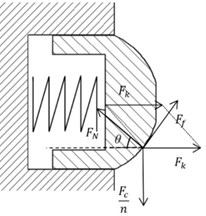Fig. 2Relative axial displacement between the plugs of different layers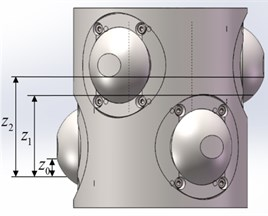Fig. 3Curves in the theory force of axially squeezing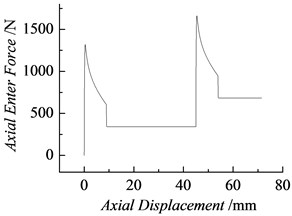Table 1Plug configuration parameters

 $R$ /mm ${d}_{0}$ /mm ${l}_{0}$ /mm $\mathrm{\Delta }{x}_{0}$ /mm $\mathrm{\Delta }{x}_{1}$ /mm ${z}_{0}$ /mm ${z}_{1}$ /mm ${z}_{2}$ /mm $K$ /N·mm-1 $\mu$ 23.288 15.852 17.599 5.000 10.000 8.852 45.000 53.852 15 0.7

## 3. Experimental studies on the pressing effect

The test system is presented in Fig. 4. The test procedures are repeated once every condition to obtain the relation curves between the axial pressure and the displacement, as shown in Figs. 5, 6, and 7, where $E1$ and $E2$ are the pressure – displacement curves of pressing into natural granite at a constant velocity with a plug spring coefficient of 15 N/mm; $E3$ and $E4$ are those of pressing into synthesized granite with a plug spring coefficient of 15 N/mm; and $E5$ and $E6$ are those of pressing into synthesized granite with a plug spring coefficient of 18 N/mm. As illustrated in Figs. 5-7, the change regularity of the axial pressure-displacement curves under the same test conditions remains unchanged. However, compared with the pressure – displacement time curves from the theoretical calculation, the test results fluctuate randomly, as shown in Fig. 8 The maximal axial pressures ${F}_{1max}$ and ${F}_{2max}$ in the process of squeezing the first and second layers of plugs into the mounting holes under different test conditions and the mean friction forces ${F}_{f1}$ while squeezing and ${F}_{f2}$ can be read from the test curves in Table 2. The four characteristic forces in the squeezing process, defined as ${F}_{1max}$, ${F}_{2max}$, ${F}_{f1}$ and ${F}_{f2}$, are short for the characteristic squeezing force.

Fig. 4System for testing the sliding friction in the squeezing process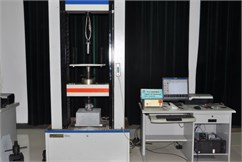a)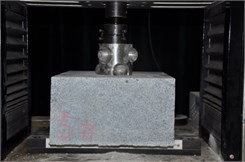b)

Fig. 5Axial pressure curves of pressing the plugs into natural rock holes (K= 15 N/mm)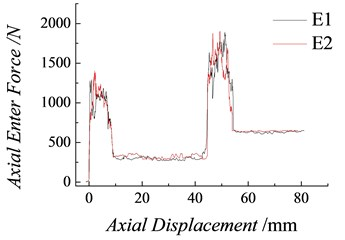Fig. 6Axial pressure curves of pressing the plugs into synthesized rock holes (K= 15 N/mm)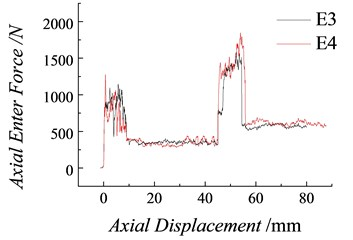Fig. 7Axial pressure curves of pressing into the synthesized rock holes (K= 18 N/mm)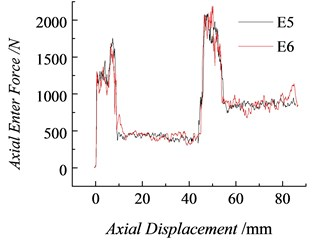Fig. 8Comparison between the theoretical and test values of the axial pressure (K= 15 N/mm)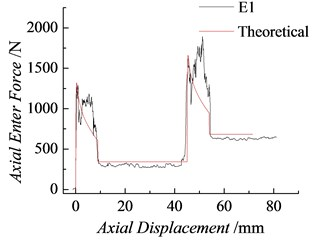In Table 2, the mean friction forces ${F}_{f1}$, ${F}_{f2}$ of the first and second layers are obtained:

3
${F}_{f1}=\frac{{\sum }_{i=1}^{{m}_{1}}{F}_{ci}}{{m}_{1}},{F}_{f2}=\frac{{\sum }_{j=1}^{{m}_{2}}{F}_{cj}}{{m}_{2}}.$

In Eq. (3), ${m}_{1}$ and ${m}_{2}$ respectively represent the number of measuring points on the first and second plateaus of the pressure test curves, and, ${F}_{ci}$ and ${F}_{cj}$ respectively represent the measured pressures on the first and second plateaus. Thus, the friction coefficient between the bulb and the rock hole wall is obtained:

4
$\mu ={F}_{f1}+{F}_{f2}/nK\mathrm{\Delta }{x}_{1},$

where n is the number of bulbs that are contacted at the same time, $K$ is the spring constant, and $\mathrm{\Delta }{x}_{1}$ is the spring compression while the bulb is being squeezed.

In Fig. 8 the theoretical and test results of the axial pressure – displacement time curves of the squeezing process are compared under Condition $E1$. As shown in the Table 3, the maximal relative error between the theoretical and test values of the squeezing force is smaller than 8 %, which indicates enhanced consistency.

Table 2Test results of the sliding friction coefficient under different test conditions

 Test conditions ${F}_{1max}$/N ${F}_{f1}$/N ${F}_{2max}$/N ${F}_{f2}$/N $\mu$ $E1$ $K=$ 15 N/mm, pressing in natural granite 1262.3 315.1 1626.2 630.2 0.70 $E2$ 1327.9 321.3 1573.4 642.6 0.71 $E3$ $K=$ 15 N/mm, pressing in the artificial sesame white granite 788.8 286.8 1310.7 573.6 0.64 $E4$ 823.2 291.9 1439.8 583.7 0.65 $E5$ $K=$ 18 N/mm, pressing in the artificial sesame white granite 1288.1 431.4 2077.9 862.7 0.96 $E6$ 1304.6 440.5 2069.1 880.9 0.98

Table 3Comparison between the theoretical and test results of the characteristic force under condition E1

 Characteristic squeezing force ${F}_{1max}$/N ${F}_{f1}$/N ${F}_{1max}$/N ${F}_{f2}$/N Mean friction coefficient $\mu$ Test value 1262.3 315.1 1626.2 630.2 0.70 Theoretical value 1320.6 342 1662.2 684 0.70 Relative error / % 4.4 7.9 2.2 7.9 /

## 4. Numerical simulation study of the pressing effect

The friction force ${F}_{f}$ between the bulb and the hole wall is correlated with the normal pressure ${F}_{n}$ on the sliding plane and the sliding contact area as follows :

5
${F}_{f}=\mu \left({F}_{n}+P{A}_{r}\right).$

The relationship is shown in Fig. 9. In the figure, ${v}_{f}$ is the velocity of movement at the boundary between adhesive friction and sliding friction. The ratio of the increment of the friction force while squeezing to the corresponding increment of the maximal squeezing force is defined as the sensitivity coefficient of the factor, which is expressed as $\omega$ and in percentage.

### 4.1. Comparison between the theoretical calculation and the numerical simulation

Through the plug configuration parameters given in Table 1, the experiment scheme $E1$, and the mean friction coefficient ($\mu =$ 0.7), a simulation model is established for the squeezing process . As shown in Fig. 10 he relative errors with the theoretical values are 0.4 % and 0.8 %, thus indicating a good agreement. The numerical simulation model and all the calculation parameters are suggested to be rational, feasible, and applicable to the present study in the change regularity of the characteristic squeezing force of the plug with different spring stiff nesses, contact frictions, and load velocities [19, 20].

### 4.2. Change regularity of the characteristic squeezing force of the plug with the contact friction

In Fig. 11 the friction coefficient on the contact surface changes from 0.5 to 1.0, with spring stiffness of 15 N/mm and squeezing velocity of 20 mm/min. In Fig. 12 the same friction coefficient, ${F}_{2max}={F}_{1max}+{F}_{f1}$, is in good agreement with the theoretical analysis result, and changes in ${F}_{1max}$, ${F}_{2max}$ with the friction coefficient are nonlinear. Fig. 13 shows the change curves of ${F}_{f1}$ and ${F}_{f2}$ with the friction coefficient. When $2{F}_{f1}={F}_{f2}$ and other conditions remaining unchanged, the plugging force may increase with the number of layers.

### 4.3. Change regularity of the characteristic squeezing force of the plug with the spring stiffness

Fig. 14 shows the axial pressure–displacement curves with a friction coefficient of 0.7, load velocity of 20 mm/min, spring stiffness coefficient increasing from 15 N/mm to 20 N/mm, and different stiffness coefficients. Figs. 15 and 16 respectively show the simulation results of the maximal squeezing forces ${F}_{1max}$, ${F}_{2max}$ and the friction forces ${F}_{f1}$. Based on the numerical simulation results of the characteristic squeezing force with different stiffness coefficients Fig. 14, the change regularities of the maximal squeezing forces ${F}_{1max}$, ${F}_{2max}$ and the friction forces ${F}_{f1}$ and ${F}_{f2}$ with the spring stiffness coefficient can be attained in the process of squeezing the plugs into holes in rock, increasing with the stiffness coefficient linearly.

In the range of $K=$ 15–20 N.m-1, the spring stiffness sensitivity coefficient ${\omega }_{stiff}=$ 34.1 % and the friction sensitivity coefficient ${\omega }_{fric}=$ 48.3 %. Therefore, while improving the installation and performance of the plug, any increase in the squeezing force peak should be avoided as much as possible by increasing the friction coefficient rather than the spring constant. This specific method is to increase both friction/contact area and surface roughness.

Fig. 15Change curves of with the spring constant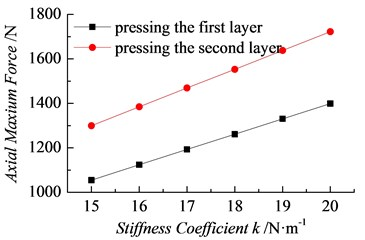Fig. 16Change curves of with the spring constant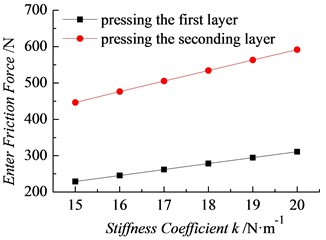Fig. 17Squeezing force with different load velocities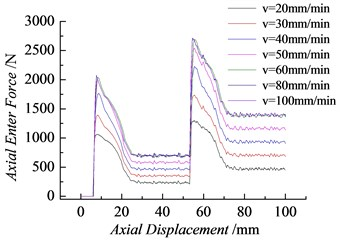Fig. 18Change curves with the load velocity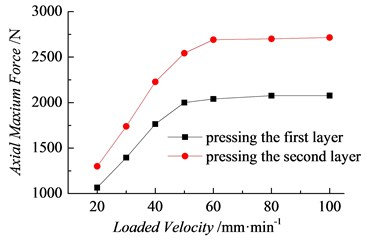### 4.4. Change regularity of the characteristic squeezing force of the plug with the load velocity

Fig. 17 shows the change regularities of the axial pressure-displacement curves attained by numerical simulation under different conditions but with a spring stiffness coefficient of 15 N/mm, plug-rock friction coefficient of 0.7, and load velocity $v=$ 20-100 mm/min. In Fig. 17, the characteristic squeezing forces ${F}_{1max}$, ${F}_{2max}$, ${F}_{f1}$ and ${F}_{f2}$ change more significantly with load velocity than with friction coefficient and spring stiffness. Figs. 18 and 19 respectively show the change regularities of the characteristic squeezing forces ${F}_{1max}$, ${F}_{2max}$, ${F}_{f1}$ and ${F}_{f2}$ with load velocity. As shown in the figure, with the increasing load velocity, the characteristic squeezing force increases with the load velocity with nonlinearity, but the increase rate gradually diminishes. When the load velocity increases to 60 mm/min, all the characteristic squeezing forces reach their peaks and then remain unchanged at $v=$ 60 mm/min. The range of load velocity $v=$ 20-60 mm.min-1 is presented in Figs. 18 and 19, and ${\omega }_{loadvelocity}=$ 65.9 %. Clearly, an appropriate increase in load velocity is suitable for improving the installation and performance of the plug. Although accelerating the plug installation in underwater construction is difficult, the loading velocity should be improved in practice as much as possible.

Fig. 19Change curves with the load velocity## 5. Conclusions

In this paper, the process of pressing the plugs into mounting holes in rock for a UBVT system is simulated numerically. The following conclusions are drawn:

1) The statics analysis, experimental test, and numerical simulation results of the squeezing process are in good agreement. The complete theories and methods established lay a solid foundation for designing the plug configuration parameters, calculating the characteristic squeezing force, developing the implementation plan, and evaluating and analyzing the plug performance.

2) The pressure-displacement curves are attained by simulation under different conditions and reveal the change regularity of the axial pressure through four steps of the squeezing process. A method is proposed to characterize the squeezing force and the characteristic squeezing forces ${F}_{1max}\text{,}$${F}_{2max}\text{,}$${F}_{f1}$ and ${F}_{f2}$ with displacement. Therefore, the change regularities and characteristics of the characteristic force with friction, spring stiffness, and load velocity are obtained.

3) A method is proposed to characterize the sensitivity coefficient of the friction coefficient, spring stiffness coefficient, and load velocity in the plug performance and plugging capacity. Therefore, the sensitivity coefficient of each of the factors influencing the performance is attained, and reference for optimizing the plug configuration parameters and developing proper process measures is provided.